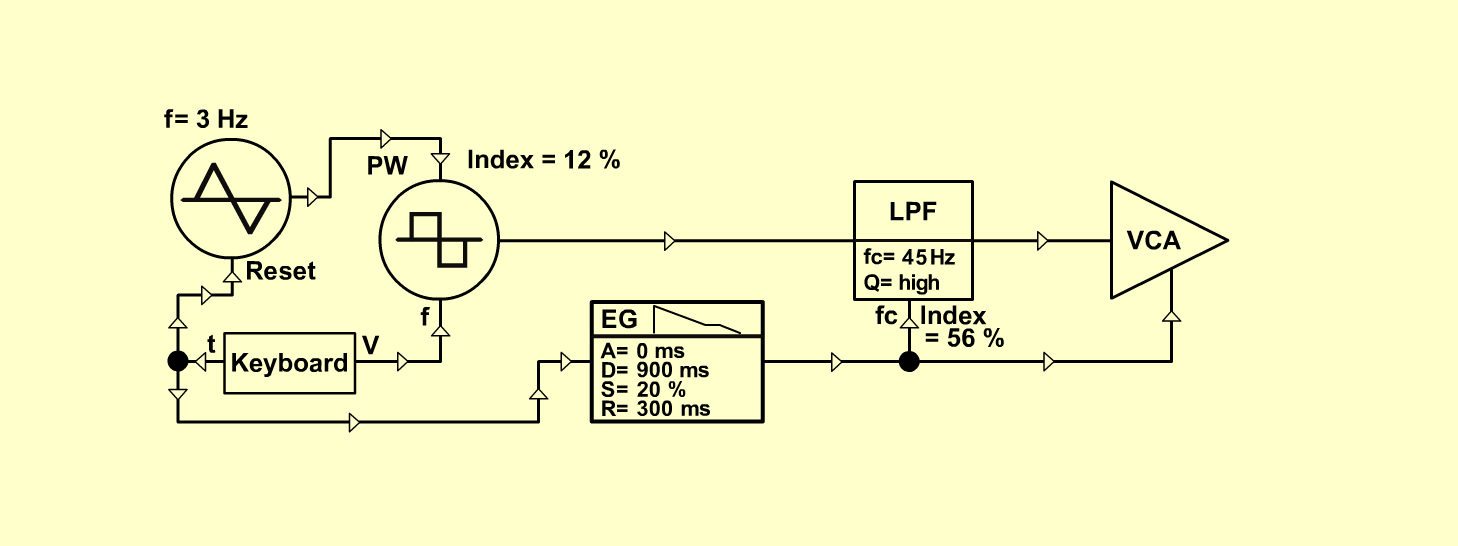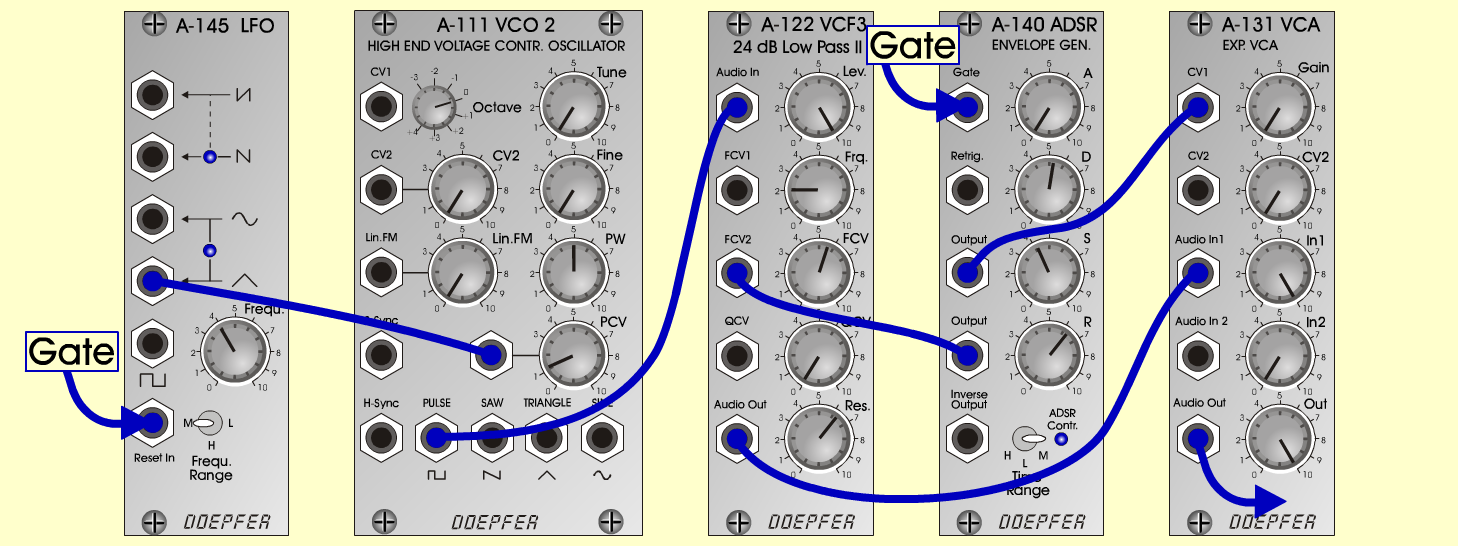Axel_JS y n t hD o e p f e r A - 100 Connections: Settings: A-145 (Tri)  <=>  A-111 (PCV) A-111 (Pulse)  <=>  A-122 (Audio In) A-122 (Audio Out)  <=>  A-131 (Audio In) A-140 (Output/1)  <=>  A-122 (CV 2) A-140 (Output/2)  <=>  A-131 (CV1) A-131 (Audio Out)  <=>  Amplifier KB (Pitch)  <=>  A-111 (CV1) KB (Gate)  <=>  A-140 (Gate) A-111 ( PW = 5, PCV = 1.2) A-122 (Level = 10, Freq = 2, FCV  = 5.6, Res = 5.7) A-131 (Gain = 0, Audio In 1 = 10,  Audio Out = 10) A-140 (A = 0, D = 5.3, S = 4.2, R = 6.3, Range = M) A-145 (Frq = 4, Range = M) Notes: This sound was patched spontaneously from Axel, when I visited him last month. Josef Mueller Axel Jungkunst, November 2001Sound samples Axel_J Piece.mp3 Axel_J (261 Hz) Axel_J (523 Hz)